Journal DetailsFormat
Journal
First Published
09 Nov 2012
Publication timeframe
4 times per year
Languages
EnglishOpen Access

# Usefulness of the CPTU method in evaluating shear modulus G0 changes in the subsoil

###### Accepted: 17 Mar 2021
Journal DetailsFormat
Journal
First Published
09 Nov 2012
Publication timeframe
4 times per year
Languages
English

This article contains the analysis of the correlation between the cone resistance qc from CPTU tests and shear modulus G0 determined from seismic tests SDMT and SCPTU. The analysis was performed for sands located in Poland, characterised by differential grain size distribution and origin. The significant impact of the independent variables; grain size, preconsolidation stress σ’p, geostatic vertical stress σ’v0 and relative density index on the dependencies analysed, were examined in three stages. Firstly, a general relationship between the cone resistance and shear modulus G0 was established; in the second stage, an analysis was carried out in selected groups of subsoil; and in the third stage, the influence of other independent variables was taken into account. In each stage, the functional form of the dependency was determined, and their statistical significance was assessed throughout coefficient of determination R2. For more variables, multivariable regression analysis was applied for assessment. Conducted analysis showed that the overall view of the relation between the cone resistance qc and shear modulus G0 has low evaluation of the statistical significance. This fact is consistent with the theoretical assessment of this relationship. To obtain a satisfactory assessment of this dependency, it is necessary to construct empirical equations for individual groups of soil, taking into account other independent variables; preconsolidation stress σ’p, vertical stress σ’vo and relative density index. This approach allows to assess the local correlation relationship, which is very useful during the geological project.

#### Keywords

Introduction

To design and examine the stability of many building structures, the knowledge of small strain shear modulus G0 is required. In the event of a significant variability in the construction of the subsoil found under the designed structure, it is necessary to continuously assess the changes in modulus G0 of the studied profiles. Such an assessment can be obtained from the empirical correlation between cone resistance qc from CPTU and small strain shear modulus G0. Using this correlation to predict changes in modulus G0 in the subsoil also allows for the reduction of the cost of field tests and reduction of the number of necessary tests used to directly determine modulus G0 with SDMT and SCPTU. Because of many independent variables that affect the measured parameters in the process of static penetration, a unique correlation between cone resistance qc and shear modulus G0 does not exist, just as in the case of the interrelationship between undrained shear strength from DMT and CPTU (Młynarek et al., 2018). Therefore, it is interesting to identify the factors and their quantitative impact on the correlation between cone resistance qc and shear modulus G0. This issue is the objective of this article. Due to the aforementioned large number of variables affecting cone resistance, the analysis was limited to non-cohesive soils of different genesis and preconsolidation effect.

Geological characteristics of test sites

The research was carried out in 5 locations that differed in geological history and allowed the collection of results regarding sediments formed in various depository environments and subjected to various post-sedimentation processes (Fig. 1). Five research sites located in Poland have a complex geological structure. Four of them (Gnojewo, Derkacze, Darłowo and Rzepin) are in the zone of impact of the Weichselian Glaciation, which left moraine deposits covered with fluvioglacial sands.

The grain size distribution of the fluvioglacial sands is very diverse, and it ranges from gravels to fine and silty sands. The layers are generally thin and do not exceed 1.5 m. However, outwash plain ‘sandur’ forms were formed in stages and over hundreds of years, which resulted into a certain variation in the state of these soils and had an impact on the formation of a minor preconsolidation effect in the subsoil. The overconsolidation ratio (OCR) of these soils is estimated within the range of 1–3. The OCR values were determined using the Wierzbicki (2010) method based on the results of CPTU. The obtained values were randomly compared with geological knowledge regarding the history of these soils.

Older sediments, the so-called interglacial sands found below the layer of the youngest moraine clay, were also examined in two locations (Derkacze and Darłowo). These deposits, which developed into the form of medium and fine sands, are characterised by a homogeneous layer structure and high values of OCR reaching 10. The last location, Warsaw, included structures created during the Riss Glaciation. As the examined fluvioglacial deposits in this region rested on moraine clay, they were geologically normally consolidated. In this case, certain effects of ageing (cementation) of the sediment were observed, because the deeper layers showed OCR values characteristic for preconsolidated soils (OCR around 6).

Theoretical foundations of the correlation between cone resistance qc and shear modulus G0

The empirical correlation between cone resistance and shear modulus G0 is built on the functional parameters that describe two different processes. One process is the process of static penetration, the other one is the course and registration of a seismic wave in the subsoil. The static penetration process is expressed with Eq. (1) (Młynarek, 2007; Młynarek et al., 2018): $F(Ps,qc,Vp,Q1,Q2)=0$F\left( {{P_s},{q_c},{V_p},{Q_1},{Q_2}} \right) = 0 where: Ps – measured parameter of CPTU test equivalent, qc, qt – cone resistance, Vp – penetration velocity, Q1 – parameter characterising soil medium, Q2 – parameter characterising cone.

For non-cohesive soils, parameter Q2 is written as a function of multiple variables (Lunne et al., 1997; Jamiolkowski et al., 2001): $Q2=f1(x1,…,x8)${Q_2} = {f_1}\left( {{x_1}, \ldots ,{x_8}} \right) where: x1 – effective unit weight of soil, x2 – grain size characterisation, x3 – relative density of soil, x4 – grain coarseness and ageing effect, x5 – mineralogical type of grain, x6 – parameter describing stress in the soil, x7 – parameter defining shear strength (co-dependent on x1x5), x8 – preconsolidation stress or OCR.

The quality of the measured cone resistance values also depends on the measurement uncertainty associated with the used test technique (Młynarek, 2010; Lumb, 1974). The impact of the independent variables recorded in parameter Q1 on the values of cone resistance and measurement uncertainty cannot be separated (Lacasse & Nadim, 1994); hence, the change of e.g. cone geometry, penetration velocity and even the use of probes from different manufacturers (Młynarek, 2010) can lead to different records of the correlation between cone resistance and shear modulus G0. Equations (1) and (2) justify the statement that there is no unique correlation for non-cohesive soils between cone resistance and modulus G0. Many authors have documented the impact of variables x1xn on cone penetration parameters, including cone resistance (e.g. Lunne et al., 1997; Mayne, 2001).

The function that describes the course and registration of a seismic wave and creates the basis for determining shear modulus G0 is expressed by a simple Eq. (3) (Lunne et al., 1997): $G0=ρVs2${G_0} = \rho V_s^2 where: ρ – soil density, Vs – shear wave velocity.

Equation (3) is supplemented with Eq. (4), which determines the independent variables that affect shear modulus G or G0 (Hardin, 1978; Lee & Stoke, 1986): $G/G0=f2(σ′v0,e0,OCR,S,C,K,T)$G/{G_0} = {f_2}\left( {\sigma {'_{v0}},{e_0},OCR,S,C,K,T} \right) where: σ’v0 – effective vertical stress, e0 – initial void ratio, OCR – overconsolidation ratio, S – degree of saturation, C – grain characteristics, S – soil structure, T – temperature.

Methodology used to obtain the data

Measurement uncertainty, as in CPTU, has an impact on the determined value of G0. These uncertainties are related to the measurement technique and seismic wave registration. Research by Foti et al. (2006) showed high recurrence of seismic wave registration in the replication test for one type of the SDMT dilatometer. A separate issue is the conformity assessment of designated moduli G0 if the test is performed with two different devices. Such situation took place in the conducted research.

During the research, a cone manufactured by AP vd Berg with an seismic module with a single geophone and a Studio Marchetti dilatometer with a seismic module with a pair of geophones located 0.5 m apart were used. To determine the time of arrival of the wave in the case of SCPTU, the pseudo-interval and cross-correlation methods were used. In the case of SDMT, the true interval method and phase shift analysis were applied (ASTM Standard, 2008). Examples of the conducted analysis of the arrival time of the wave are presented in Fig. 2. Determining the time of arrival of the wave allows calculation of the small strain shear modulus G0 according to the Eq. (3). These results, supplemented with the values of CPTU parameters (qc, fs, u2), formed the basis of the data set used in the analysis (Figs. 3 and 4).

The seismic measurements have been done every 0.5 m or 1.0 m of profile (dependently on the testing site). The CPTU data were averaged within defined geotechnical layers and were correlated with the seismic measurements carried out within each particular layer and depth. Data groups from this set, which were correlated with the depths from which samples were taken for laboratory analyses, were selected for further examination.

The statistical significance of differences between the designated moduli G0 from both devices can be examined by analysing the trend of modulus G0 changes with depth (Lumb, 1974). As far as sediments are concerned, Młynarek et al. (2006) showed that the trend of modulus G0 changes with depth is rectilinear, and the trend equation coefficients do not significantly differ in terms of statistics. This type of analysis was carried out for the examined sands and is presented in Fig. 5. The obtained results prove that SDMT and SCPTU give statistically non-differing assessments of how small strain shear modulus G0 changes with depth (Fig. 5).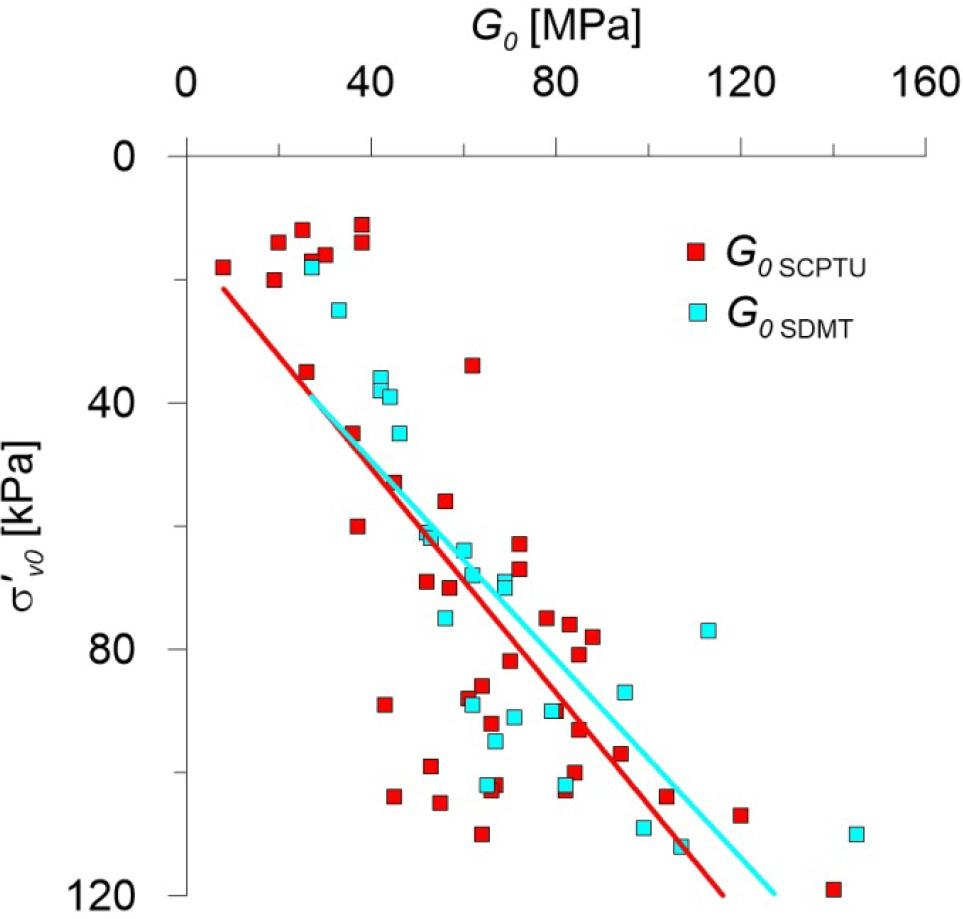Trend of changes in shear modulus G0 with depth for SCPTU and SDMT performed in normally consolidated medium sands (data set from Derkacze and Gnojewo test sites).

Figure 6 additionally shows the distribution of population of shear moduli G0 from SCPTU and SDMT in regard to cone resistance qc, which includes variability of soil grain size, vertical stress, thickness and diversity of origin of non-cohesive soils tested. Figure 6 shows that the modulus values obtained from both studies are located along almost identical trend lines. This fact proves that both techniques consistently register the impact of the parameters listed in Eq. (4) on the variability of modulus G0. The obtained result also justifies the possibility of using the value of G0 obtained from both tests to create a correlation between the shear modulus and cone resistance.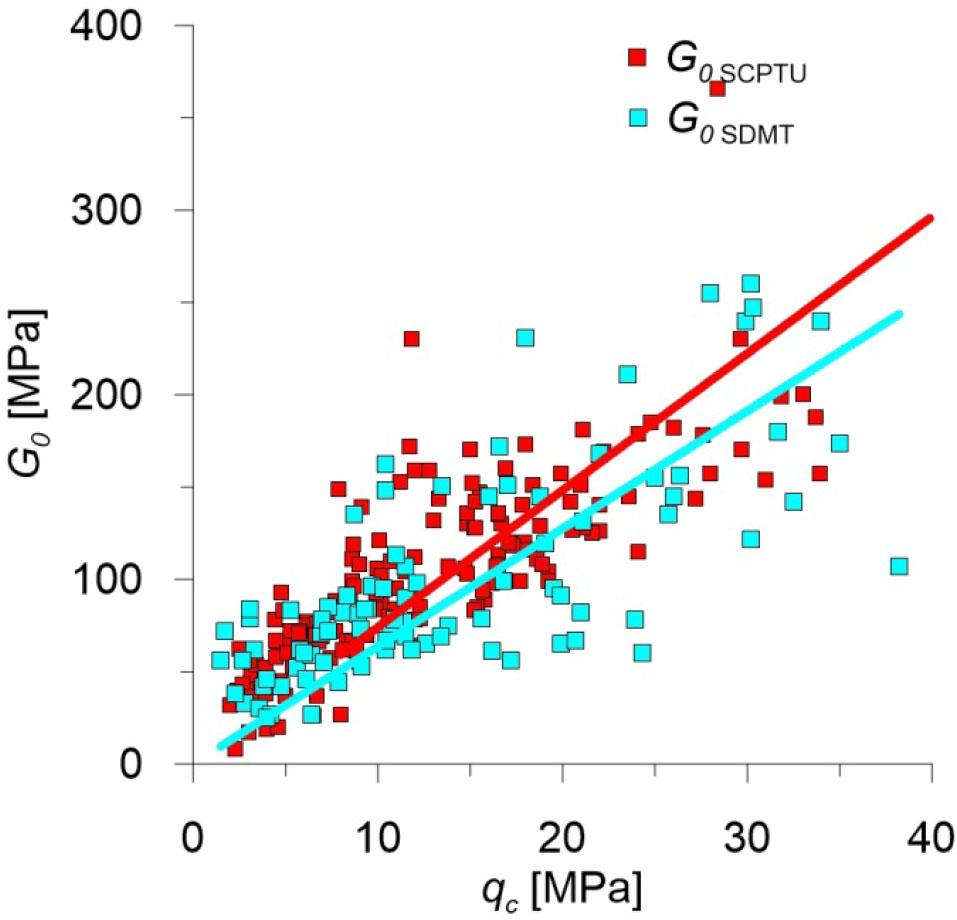Distribution of shear modulus population G0 from SCPTU and SDMT with respect to cone resistance qc (data set at all test sites).

Several solutions for Eq. (4) are known in the literature. An example of such correlation is Eq. (5) by Jamiolkowski et al. (1995): $G0=480e−1.43σ′v00.22σ′h00.22pa0.66${G_0} = 480{e^{ - 1.43}}\sigma _{v0}^{'0.22}\sigma _{h0}^{'0.22}p_a^{0.66} where: pa – atmospheric reference stress in the same unit as G0, e – void ratio, σ’v0 – effective vertical stress, σ’h0 – effective horizontal stress.

In Eqs. (1) and (2), and (3) and (4), the same independent variables related to the ground are found. This fact is an accurate justification for the purpose of constructing the correlation for non-cohesive soils between cone resistance and shear modulus G0. However, an interesting question remains: how do the variables appearing in Eqs. (2) and (4) affect the correlation between cone resistance and shear modulus G0? To answer this question, as mentioned in point 2, research was carried out in several locations in Poland. The results allowed an analysis taking into account the variables that define grain characteristic, relative density and soil structure. These variables are associated with different sediment genesis and preconsolidation effect. Preconsolidation stress σ’p, was used to describe the preconsolidation effect, while grain characteristics were taken into account by performing an analysis in four groups of non-cohesive soils, both normally consolidated and preconsolidated silty sands, fine sands, medium sands and gravels. The second separately analysed issue was the identification and assessment of the significance of the impact of the variables present in Eq. (4) on shear modulus G0. This analysis was performed again in various groups of non-cohesive soils, and the following variables were taken into account: the degree of thickness instead of the initial void ratio, preconsolidation stress σ’p and effective vertical stress σ’v0.

Analysis of the results
Analysis of the correlations between cone resistance qc and shear modulus G0

An important issue for using the correlations between shear modulus G0 and cone resistance is the statistical assessment of the significance of this correlation. A certain difficulty for this assessment is the fact that some variables from Eqs. (2) and (4) are not written in a discrete form, e.g. ageing, cementation, macrostructure, although they have a significant impact on the value of the coefficient of determination R2. For this reason, the analysis of the correlation between modulus G0 and cone resistance was carried out in stages. A set of 238 data from 6 locations was used in the analysis. The first step in the analysis was to examine the basic correlation qcG0, for the entire population (Fig. 7).

The correlation between modulus G0 and cone resistance qc for the entire population is logarithmic, but its statistical significance is not high. The second step in the analysis was to identify the impact of stress history on the analysed correlation. To this end, using general geological knowledge regarding deposition environments and calculated OCR values, the soils were grouped into normally consolidated and overconsolidated soils. In this step of the analysis, a significant improvement in the coefficient of determination R2 was obtained for normally consolidated soils (Fig. 8).The correlation between modulus G0 and cone resistance qc taking into account the division into normally consolidated (blue) and overconsolidated (red) soils.

To test the effect of grain roughness, which is defined by variable x2 in Eq. (2), on the relationship between the cone resistance qc and shear modulus Go in Fig. 9 shows the test results of Norwegian Geotechnical Institute (Lunne et al., 2003). This research covered deposits of fluvial sands from Holmen (Norway). These deposits are normally or lightly overconsolidated from a geological point of view. Despite the similar granulometric and mineral composition and thickness, these soils significantly differ in origin and degree of roughness of grains from those found in Poland.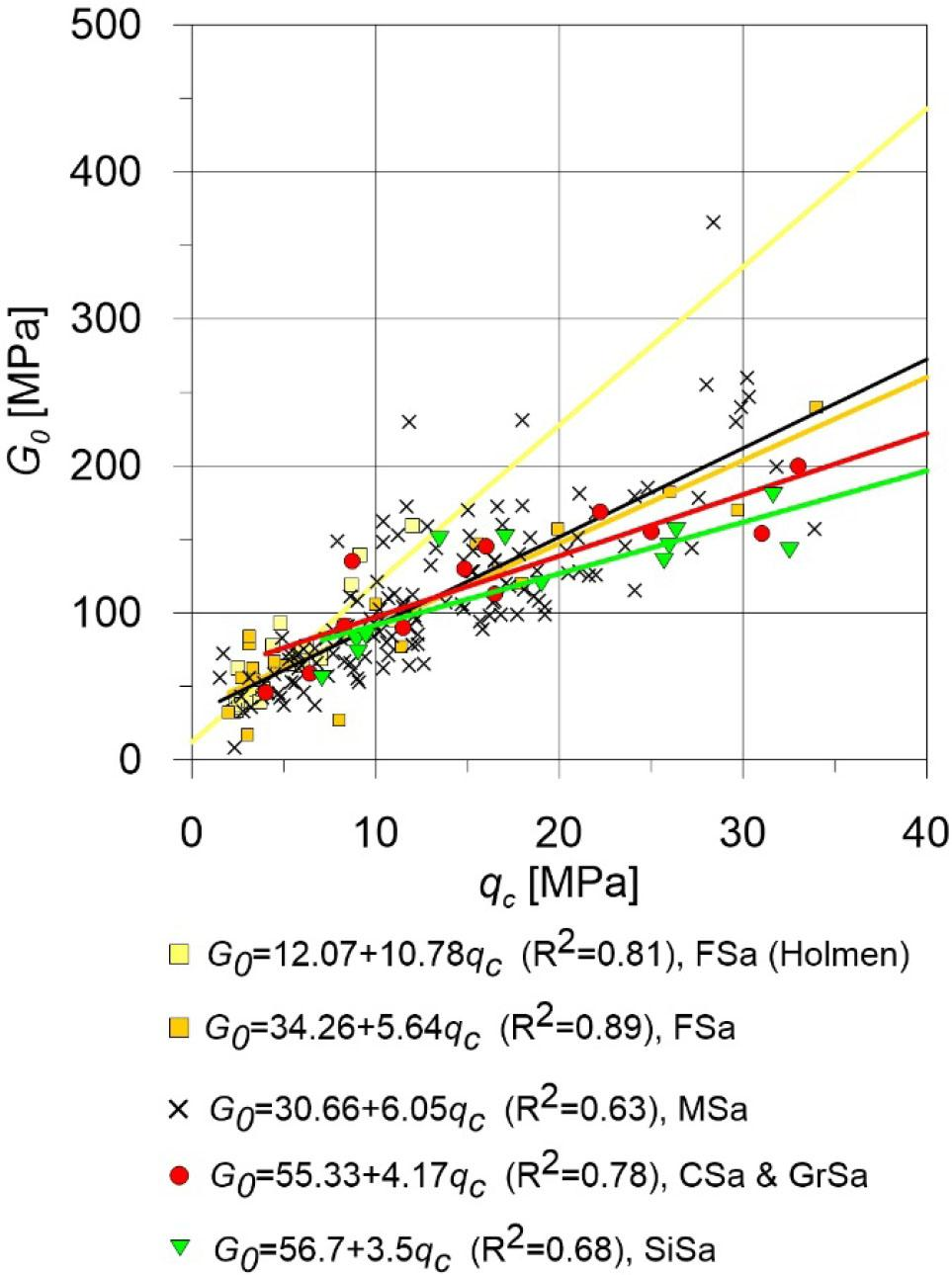The correlation between modulus G0 and cone resistance qc for normally consolidated soils taking into account the type of soil.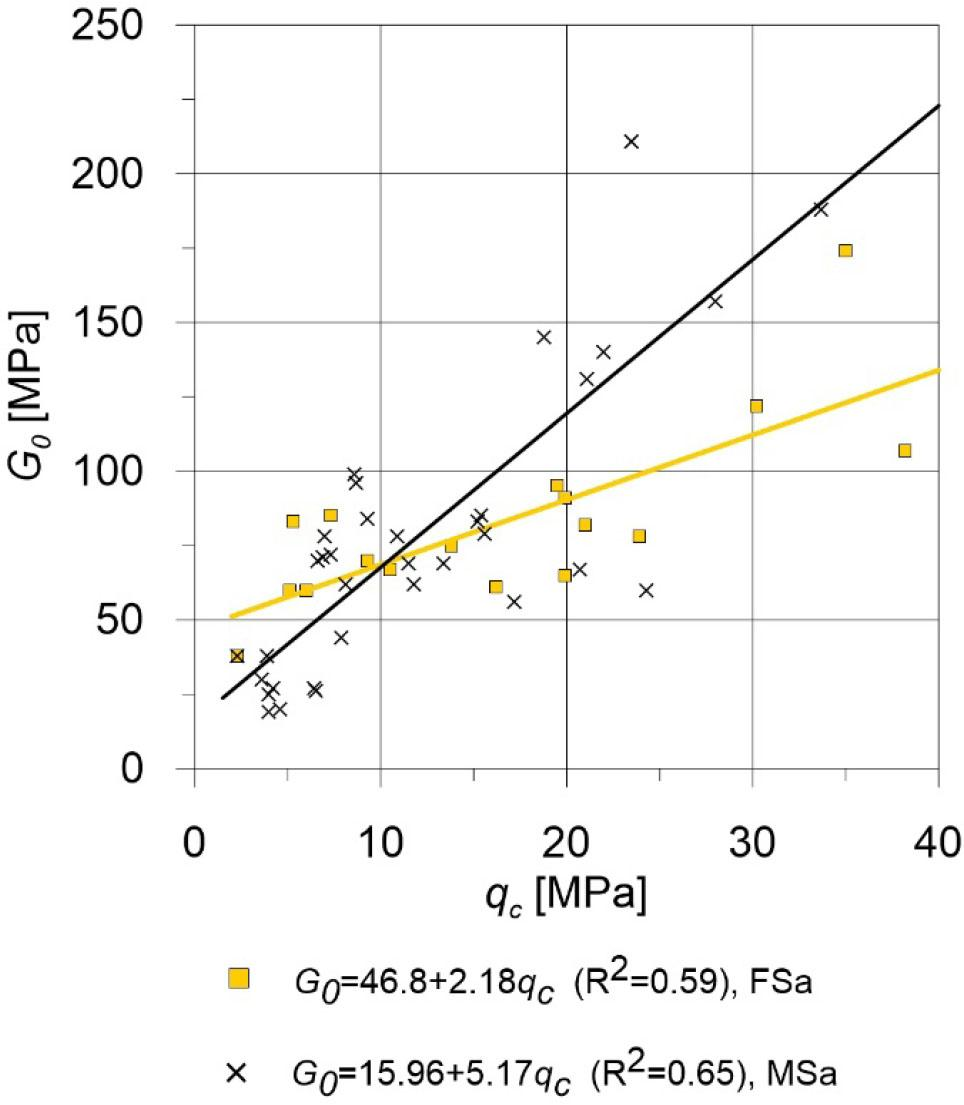The correlation between modulus G0 and cone resistance qc for overconsolidated soils taking into account the type of soil.

The third step of the analysis additionally considered the impact on the correlation between the G0 modulus and qc cone resistance variables, which define the granulometric composition of the studied soils and their origin. For this purpose, the data were divided into 5 groups: silty sands (SiSa), fine sands (FSa), fluvial fine sands (FSa Holmen), medium sands (MSa), coarse sands and gravels (CSa and GrSa) (Fig. 9). The mentioned types of soils were determined based on the laboratory tests performed on 115 soil samples.

To determine the unit weight of soils in the subsoil, empirical dependencies between the cone resistance qc, and g0 (unit weight of soil) were applied, taking into account the type of soil and relative density index – Dr (Młynarek at al., 2019).

Figure 9 shows that the normally consolidated fine sands from Holmen are located on the diagram in different part of the plot than the normally consolidated fine sands from Poland. It could be because of a different origin and some diferences between them in angularity. Fluvioglacial sediments from Poland are more sharp-edged; however, the quasi preconsolidation effect present in the Holmen test site soils makes them occupy the upper part of the graph in Fig. 9. This fact is confirmed by the impact of this variable expressed in Eq. (2) for the mentioned correlation. In most cases, the used division allowed to obtain the value of the determination coefficient confirming the significant impact of grain size on the analysed correlation.

The first and second stage of the analysis showed that the dominant role in the analysed correlation between shear modulus G0 and cone resistance qc is played by both the stress history, which modifies the state of stress, and the origin and grain size distribution of soils. In the fourth stage, an attempt was made to create the most versatile model possible determining shear modulus G0 using multivariable regression analysis. Shear modulus G0, was adopted as a dependent variable, and cone resistance qc preconsolidation stress s’p and soil type and OCR) as independent variables.

Soils with different grain sizes were grouped into normally consolidated and overconsolidated soils in the first part of this analysis. In the case of normally consolidated soils, the analysis includes both the division of soils into individual types and the absence of such a division. The preconsolidation stress s’p was calculated according to Eq. (6) (Wierzbicki, 2010): $σ′p=0,953e0,007Qt$\sigma {'_p} = 0,953{{\rm{e}}^{0,007{Q_t}}} where e is the Euler number, Qt =(qt-sv0)/sv0.

The following correlations were obtained as a result of the analysis:

fine sands NC: $G0=26.197+4.146qc+0.103σ′p${G_0} = 26.197 + 4.146{q_c} + 0.103\sigma {'_p}

R2=0.85, n=43

medium sands NC: $G0=12.238−1.816qc+0.463σ′p${G_0} = 12.238 - 1.816{q_c} + 0.463\sigma {'_p}

R2=0.71, n=128

silty sands NC: $G0=27.316−0.089qc+0.239σ′p${G_0} = 27.316 - 0.089{q_c} + 0.239\sigma {'_p}

R2=0.83, n=14

coarse sands and gravels NC: $G0=73.445+2.298qc+0.059σ′p${G_0} = 73.445 + 2.298{q_c} + 0.059\sigma {'_p}

R2=0.60, n=11

fine sands OC: $G0=46.698+2.232qc−0.002σ′p${G_0} = 46.698 + 2.232{q_c} - 0.002\sigma {'_p}

R2=0.51, n=17

medium sands OC: $G0=17.424+3.460qc+0.061σ′p${G_0} = 17.424 + 3.460{q_c} + 0.061\sigma {'_p}

R2=0.68, n=25

where: G0 [MPa], qc [MPa], s’p [kPa].

It is worth to note that the value of s’p is obtained from the empirical correlation, and in this case, maybe strongly influenced by the local conditions. However, the use of s’p values gives still higher statistical significance level of the correlation with G0 than s’v0 in the case of analysed set of data.

The obtained values of the determination coefficient R2 prove that the multivariate dependency model quite well assesses the shear modulus G0 prognosis based on the cone resistance and preconsolidation stress for individual soil groups.

The purpose of constructing a multivariate model is also demonstrated by the use of the correlation proposed by Młynarek et al. (2012) for overconsolidated clayey sand from Poland – Eq. (13): $G0=92.16+3.21qc+16.8OCR+0.103qc2−−2.42OCRqc+10.21OCR2$\matrix{{{G_0} = 92.16 + 3.21{q_c} + 16.8OCR + 0.103q_c^2 - } \hfill \cr { - 2.42OCR{q_c} + 10.21OC{R^2}} \hfill \cr }

R2=0.42, n=48

Figure 11 shows the location of the shear modulus G0 values, which were calculated in accordance with this correlation. The location of the value G0 in the lower zone outside the area determined by the values obtained on the basis of Eqs. (7–12) also proves the need to construct the so-called local correlations between the shear modulus G0 and cone resistance, taking into account the variables adopted in the regression model.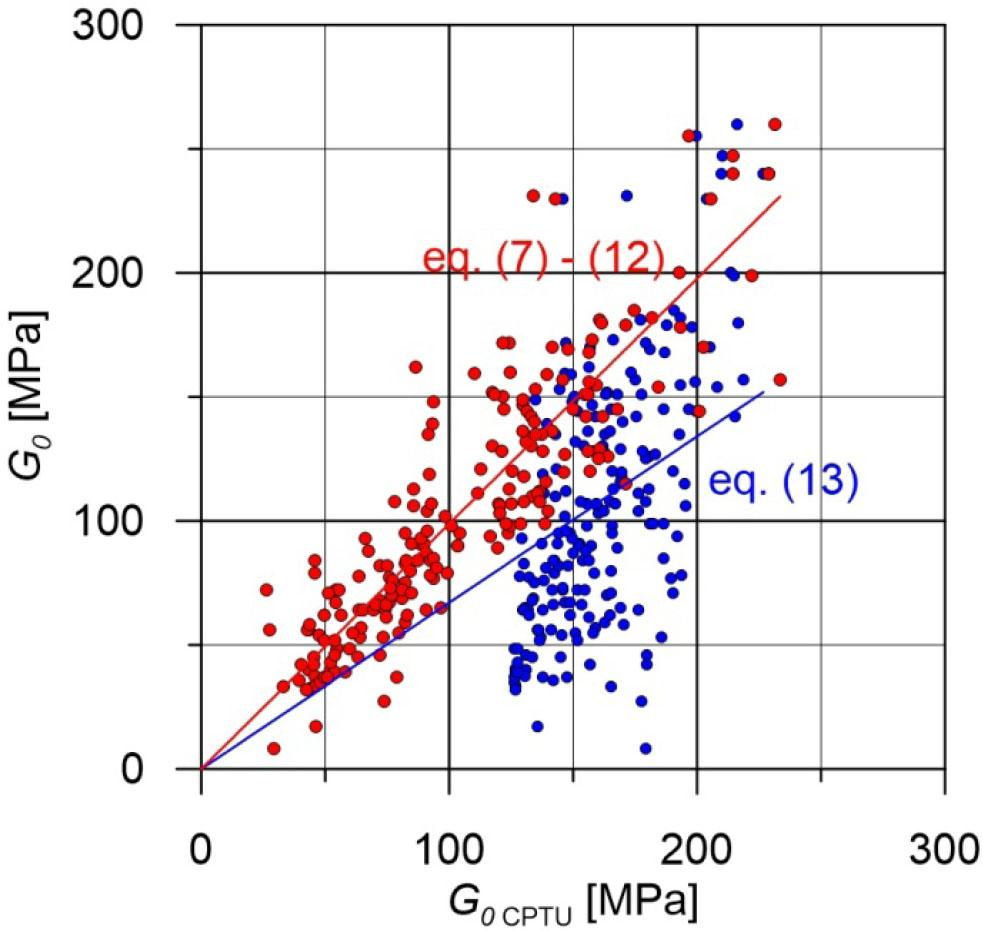Comparison of G0 values measured and calculated on the basis of Eqs. (7–12) (red) and Eq. (13) (blue).
Identification of factors affecting shear modulus G0 variability

The assessment of the impact of soil physical parameters and stress in the subsoil on modulus G0 was carried out by analysing partial functions and using multivariate analysis of variance.

The impact of effective vertical stress σ’v0 on the modulus G0 variability is shown in Fig. 12. This figure proves that for the entire population of the determined values of modulus G0, i.e. for all the soils tested, the division into normally consolidated and overconsolidated soils takes place again. Only in the zone of small G0 modulus values and small measurement depth (small values σ’v0), the effect of overconsolidation is not significant. This is documented by area A in Fig. 12. This effect was also found for the correlation between G0 and cone resistance qc. This problem has been presented in point 5.1.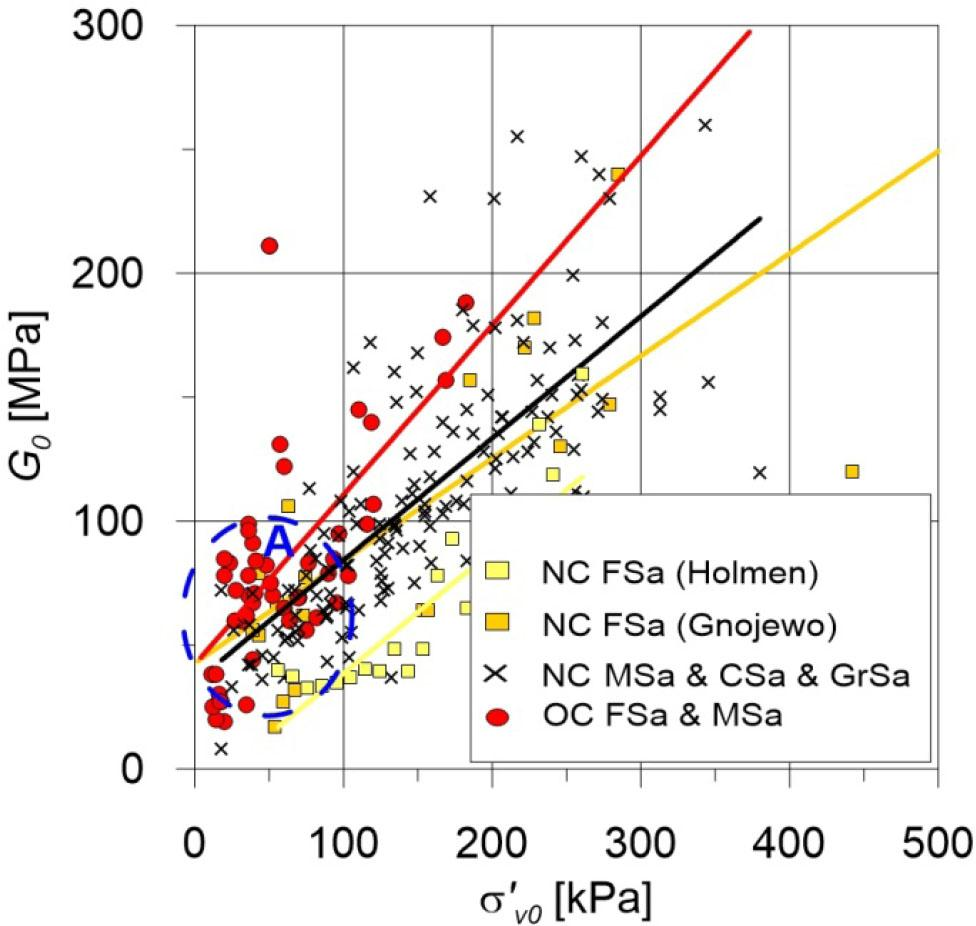The correlation between G0 and the vertical stress (s’v0) for overconsolidated (OC) (red) and normally consolidated (NC) soils distinguishing between fine sands (FSa) from Gnojewo test site (dark yellow) and Holmen test site (light yellow) and medium sands (MSa), coarse sands (CSa) and sandy gravels (GrSa).

Another analysed partial function was the correlation between modulus G0 and preconsolidation stress σ’p. Figure 13 very clearly documents the impact of preconsolidation stress, i.e. also the origin of the studied soils, on the G0 modulus values. Analysing the partial function G0 = f (σ’v0), it can be seen that soils with similar granulation, but deposited in fluvial environments and currently occurring under the sea surface (Holmen test site), can be clearly distinguished from glaciofluvial sediments deposited in the sandur environment (e.g. Gnojewo test site). A different conclusion is obtained from the analysis of the correlation between G0 modulus with preconsolidation stress σ’p. This fact probably confirms the impact of the variable on G0 modulus, which describes the macrostructure of these deposits in Eq. (4). Another reasons can also be that σ’p is quite uncertain because it is based on another empirical correlation.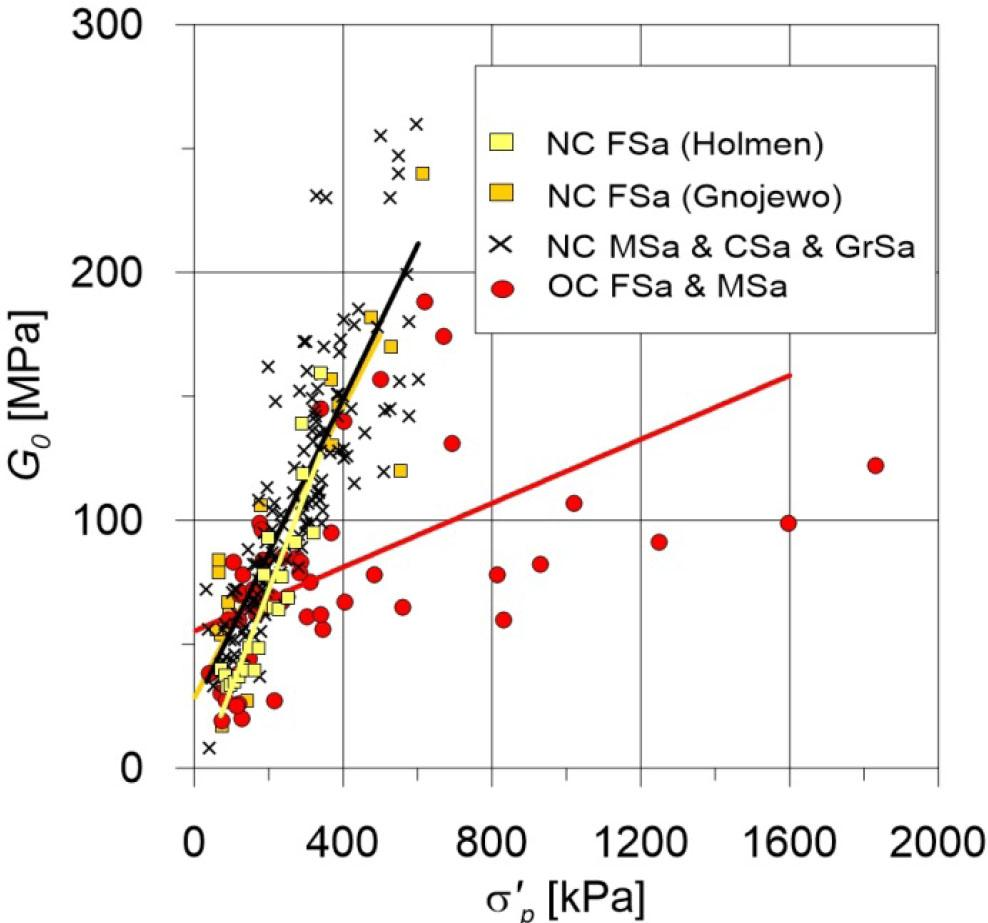The correlation between G0 and the preconsolidation stress (σ’p) for overconsolidated (OC) (red) and normally consolidated (NC) soils distinguishing between fine sands (FSa) from Gnojewo test site (dark yellow) and Holmen test site (light yellow) and medium sands (MSa), coarse sands (CSa) and sandy gravels (GrSa).

Another analysed partial function is the correlation between modulus G0 and density ratio Dr. Density ratio was determined from SCPTU using the Jamiolkowski et al. (1995) method, taking into account the mean stress σ’m0. Scope of variability of relative density index was shown in Fig. 14. $Dr=1C2h(qcC0(σ′m0)C1)${D_r} = {1 \over {{C_2}}}{\rm{h}}\left( {{{{q_c}} \over {{C_0}{{\left( {\sigma {'_{m0}}} \right)}^{{C_1}}}}}} \right) where: σ’m0 – average geostatically even stress (2 σ’h0 + σ’v0)/3; correction empirical coefficients C0= 24.94; C1 = 0.46; C2 = 2.96, Dr expressed in %, qc and σ’v0 in bars.

The impact of soil origin is less exposed (Fig. 14) in correlations between modulus G0 and density ratio. To examine the combined effect of the analysed variables on modulus G0, multivariate analysis of variance was used again. Normally consolidated and overconsolidated fine sands were included in one group in order to obtain greater variability in preconsolidation stress σ’p. The following correlations were obtained for individual soil groups Eqs. (15–18):

fine sands NC and OC: $G0=−17.559+112.097Dr+0.326σ′v0+0.001σ′p${G_0} = - 17.559 + 112.097{D_r} + 0.326\sigma {'_{v0}} + 0.001\sigma {'_p}

R2 = 0.67

medium sands NC and OC: $G0=−3.879+42.351Dr+0.397σ′v0+0.109σ′p${G_0} = - 3.879 + 42.351{D_r} + 0.397\sigma {'_{v0}} + 0.109\sigma {'_p}

R2 = 0.71

coarse sands and gravels NC: $G0=85.219−10.604Dr−0.147σ′v0+0.240σ′p${G_0} = 85.219 - 10.604{D_r} - 0.147\sigma {'_{v0}} + 0.240\sigma {'_p}

R2 = 0.52

silty sands NC: $G0=−19.569+120.713Dr+0.249σ′v0+0.024σ′p${G_0} = - 19.569 + 120.713{D_r} + 0.249\sigma {'_{v0}} + 0.024\sigma {'_p}

R2 = 0.83

The analysis of partial regression coefficients shows that the impact of individual variables on modulus G0 variability is similar, but the level of density ratio is dominant. The proportion of uncontrolled variables, e.g. macrostructure, grain coarseness, is much smaller than the correlation between shear modulus G0 and cone resistance qc. This is demonstrated by high values of the total determination coefficient R2, which ranges between 0.54 and 0.84 for individual soil groups.

The value of coefficient R2 rapidly declines to 0.44 for NC soils and 0.43 for OC soils if we construct the general dependency between G0 modulus and relative density index – Dr, taking into account only the effect of subsoil preconsolidation (Fig. 14). This fact justifies the necessity to review this dependency in different types of soil (Eqs. 15–18). The result of this analysis is consistent with the general assessment of dependency between cone resistance qc and G0 modulus, commented in point 5.1 and presented in Fig. 7.

Conclusions

The conducted tests show that the correlation between cone resistance from the SCPTU method and shear modulus G0 is an effective way to determine changes in this modulus in a non-cohesive subsoil. However, the documented effect of many variables on this correlation results in the need to construct this correlation for individual soil groups because of their grain size and their origin. Taking these variables into account makes it possible to obtain a statistically very favourable assessment of the correlation between cone resistance and shear modulus G0. Equations (7–12) can be recommended for geotechnical design, as long as they are applied locally. The effect of soil genesis can be taken into account to some extent using the OCR or effective preconsolidation stress σ’p. Test results also confirmed the strong correlation between modulus G0 determined from SCPTU and SDMT and soil physical parameters and stress in the subsoil. It is necessary to emphasise that still the best solution is to carry out SCPTU/SDMT to have less uncertainties in G0.

ASTM, Standard Test Methods for Downhole Seismic Testing, D 7400-08, West Conshohocken, PA, United States, 2008.ASTMStandard Test Methods for Downhole Seismic Testing, D 7400-08West Conshohocken, PA, United States2008Search in Google Scholar

Foti, S., Lancellotta, R., Marchetti, D., Monaco, P. Totani, G. (2006). Interpretation of SDMT tests in a transversely isotropic medium. In: 2nd International Flat Dilatometer Conference, Washington D.C. USA, 275–280.FotiS.LancellottaR.MarchettiD.MonacoP.TotaniG.2006Interpretation of SDMT tests in a transversely isotropic mediumIn:2nd International Flat Dilatometer ConferenceWashington D.C. USA275280Search in Google Scholar

Hardin B.O. (1978). The nature of stress-strain behaviour for soils. In: ASCE Geotechnical Division Specialty Conference on Earthquake Engineering and Soil Dynamics, Pasadena, 1, 3–90.HardinB.O.1978The nature of stress-strain behaviour for soilsIn:ASCE Geotechnical Division Specialty Conference on Earthquake Engineering and Soil DynamicsPasadena1390Search in Google Scholar

Jamiolkowski M., Lancellotta R., Lo Presti D.C.F. (1995). Remarks on the stiffness at small strain of six Italian clays. In: International Symposium on Pre-failure Deformation Characteristics of Geomaterials, Hokkaido ’94, v.2, 817–836.JamiolkowskiM.LancellottaR.Lo PrestiD.C.F.1995Remarks on the stiffness at small strain of six Italian claysIn:International Symposium on Pre-failure Deformation Characteristics of Geomaterials, Hokkaido ’942817836Search in Google Scholar

Jamiolkowski, M., Lo Presti, D.C.F., Manassero, M. (2001). Evaluation of relative density and shear strength of sands from cone penetration test (CPT) and flat dilatometer test (DMT). ASCE Geotechnical Special Publication No. 119, 201–238.JamiolkowskiM.Lo PrestiD.C.F.ManasseroM.2001Evaluation of relative density and shear strength of sands from cone penetration test (CPT) and flat dilatometer test (DMT)ASCE Geotechnical Special Publication119201238Search in Google Scholar

Lacasse S, Nadim, F. (1994). Reliability issues and future challenges in geotechnical engineering for offshore structures. In: International Conference. Behaviour of offshore structures. Boss94, Cambridge Mass.LacasseSNadimF.1994Reliability issues and future challenges in geotechnical engineering for offshore structuresIn:International Conference. Behaviour of offshore structuresBoss94, Cambridge MassSearch in Google Scholar

Lee S.H.H, Stoke K.H. (1986). Investigation of low amplitude shear wave velocity in anisotropic materials. Geotechnical Report No. GR 86-6, Civil Engineering Department, University of Texas, Austin.LeeS.H.HStokeK.H.1986Investigation of low amplitude shear wave velocity in anisotropic materialsGeotechnical Report No. GR 86-6Civil Engineering Department, University of TexasAustinSearch in Google Scholar

Lumb P. (1974). Applications of Statistics in Soil Mechanics. In: Soil Mechanics-New Horizons. Edited by Lee J, K. Newness-Batterworth, London.LumbP.1974Applications of Statistics in Soil MechanicsIn:Soil Mechanics-New HorizonsEdited byLeeJ, K.Newness-BatterworthLondonSearch in Google Scholar

Lunne T., Robertson P.K., Powell J.J.M. (1997). Cone penetration testing in geotechnical practice. E&FN Spon, London.LunneT.RobertsonP.K.PowellJ.J.M.1997Cone penetration testing in geotechnical practiceE&FN SponLondonSearch in Google Scholar

Lunne, T., Long, M. & Forsberg, C. (2003). Characterization and engineering properties of Holmen sand. Characterization and Engineering Properties of Natural Soils (1), Swets and Zeitlinger, Lisse, 1121–1148.LunneT.LongM.ForsbergC.2003Characterization and engineering properties of Holmen sandCharacterization and Engineering Properties of Natural Soils (1)Swets and ZeitlingerLisse11211148Search in Google Scholar

Mayne P.W. (2001). Stress-strain-strength-flow parameters from enhanced in-situ tests. In: International Conference on In-Situ Measurement of Soil Properties & Case Histories [In-Situ 2001], Bali, Indonesia, May 21–24, 2001.MayneP.W.2001Stress-strain-strength-flow parameters from enhanced in-situ testsIn:International Conference on In-Situ Measurement of Soil Properties & Case Histories [In-Situ 2001]Bali, IndonesiaMay 21–24, 2001Search in Google Scholar

Młynarek Z. (2007). Site investigation and mapping in urban area.” In: Geotechnical Engineering in Urban Environments, Madrid. Vol. 1. Edited by V. Cuéllar et al. Millpress Science Publishers, Rotterdam, 175–202.MłynarekZ.2007Site investigation and mapping in urban areaIn:Geotechnical Engineering in Urban Environments, Madrid1Edited byCuéllarV.Millpress Science PublishersRotterdam175202Search in Google Scholar

Młynarek Z. (2010). Quality of in situ and laboratory tests contribution to risk management, In: 14th Danube-European Conference on Geotechnical Engineering, Bratislava, Slovakia, 2–4.06.2010.MłynarekZ.2010Quality of in situ and laboratory tests contribution to risk managementIn:14th Danube-European Conference on Geotechnical EngineeringBratislava, Slovakia2–4.06.2010Search in Google Scholar

Młynarek Z., Gogolik S., Marchetti S., Marchetti D. (2006). Suitability of SDMT test to assess geotechnical parameters of post-flotation sediment. In: 2nd International Flat Dilatometer Conference, Washington D.C. USA, 148–153.MłynarekZ.GogolikS.MarchettiS.MarchettiD.2006Suitability of SDMT test to assess geotechnical parameters of post-flotation sedimentIn:2nd International Flat Dilatometer ConferenceWashington D.C. USA148153Search in Google Scholar

Młynarek Z., Wierzbicki J., Stefaniak K. (2012). Deformation characteristics of overconsolidated subsoil from CPTU and SDMT tests. In: Geotechnical and Geophysical Site Characterization 4, Edited by R. Q. Coutinho & P.W. Mayne. Taylor & Francis Group, London, 1189–1193.MłynarekZ.WierzbickiJ.StefaniakK.2012Deformation characteristics of overconsolidated subsoil from CPTU and SDMT testsIn:Geotechnical and Geophysical Site Characterization 4Edited byCoutinhoR. Q.MayneP.W.Taylor & Francis GroupLondon11891193Search in Google Scholar

Młynarek Z., Wierzbicki J. & Stefaniak K. (2018). Interrelationship between undrained shear strength from DMT and CPTU tests for soils of different origin. Geotechnical Testing Journal, 41(5), 890–901.MłynarekZ.WierzbickiJ.StefaniakK.2018Interrelationship between undrained shear strength from DMT and CPTU tests for soils of different originGeotechnical Testing Journal415890901Search in Google Scholar

Młynarek Z., Wierzbicki J., Stefaniak K. (2019). Usefulness of the CPTU method in evaluating unit weight of soil changes in the subsoil. Inżynieria Morska i Geotechnika. 6, 294–302.MłynarekZ.WierzbickiJ.StefaniakK.2019Usefulness of the CPTU method in evaluating unit weight of soil changes in the subsoilInżynieria Morska i Geotechnika6294302Search in Google Scholar

Wierzbicki J. (2010). Evaluation of subsoil overconsolidation by means of in situ tests at aspect of its origin. University of Life Sciences in Poznań Publishing, 410, Poznań, Poland.WierzbickiJ.2010Evaluation of subsoil overconsolidation by means of in situ tests at aspect of its originUniversity of Life Sciences in Poznań Publishing410Poznań, PolandSearch in Google Scholar

• #### Usefulness of the CPTU method in evaluating shear modulus G0 changes in the subsoil

Recommended articles from Trend MD